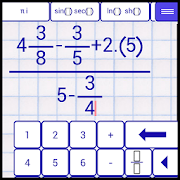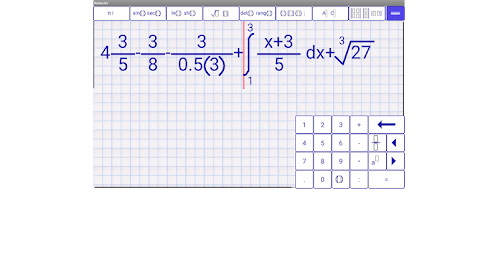Everyone
2,799★★★★★ Scientific calculator for engineers and mathematicians.

FEATURES:
Intuitive input and editing.
Saving expressions. Save as PNG.
On editor, you can use select, copy, cut, paste for expressions.
Pinch-To-Zoom
Showing result as decimal or fraction.
Undo and redo.
Choosing font.

SUPPORTED FUNCTIONS:
● Functions Graphic.
● Calculation of mixed, improper fraction and recurring decimal (repeating decimal, periodic numbers).
● Periodic number to fraction
● Fraction to decimal, Decimal to fraction
● Operations with matrices, vectors and complex numbers.
● Trigonometric functions: sin, cos, tan, ctan.
- The calculation of trigonometric functions in degrees and radians. Use symbol ° for degrees, symbol ' for minute, symbol '' for second.
● Inverse trigonometric functions: asin, acos, atan, actan
● Secant (sec), Cosecant (csc).
● Logarithms: ln, lg, log
- Ln: natural logarithm.
- Lg: common logarithm.
● Constants: π, e
● Hyperbolic functions: sh, ch, th, cth
● The square root , the root of n-th degree ⁿ√, Module | a |, Signum (sign), exponentiation aⁿ.
● Combination, Arrangement, Factorial (!)
● The sum and product elements of the sequence: Σ, П
● Brackets: ( ) [ ] { }
● Base conversion of numbers and operations with different base (binary, ternary, quintal, octal, hexadecimal, decimal, base n).
● Calculations of limit, definite integral.
● Percent (%)
● Least (Lowest) Common Multiple (LCM) for fraction and integer numbers
● Greatest Common Divisor (GCD) for fraction and integer numbers
● Matrices determinant, rang, inverse, addition, subtraction, multiplication, division
● Complex numbers addition, subtraction, multiplication, division

All in one calculator. Lightweight and simple calculator. Easy to use and understand expressions. Works offline. Advanced engineering calculator. It will help you doing homework study for school. It will make easy calculations from algebra and physic.
Collapse

Review Policy
3.9
2,799 total
5
4
3
2
1

What's New

Bugs fixed
Collapse

Updated
October 12, 2019
Size
4.4M
Installs
500,000+
Current Version
4.3.2
Requires Android
4.1 and up
Content Rating
Everyone
Permissions
Offered By
Scientific Software Team
Developer
Globus Center J.J. / 609